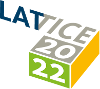#The 39th International Symposium on Lattice Field Theory (Lattice 2022)

Aug 8 – 13, 2022
Hörsaalzentrum Poppelsdorf
Europe/Berlin timezone

## Resummed lattice QCD equation of state at finite baryon density: strangeness neutrality and beyond

Aug 11, 2022, 10:40 AM
20m
CP1-HSZ/0.011 (CP1-HSZ) - HS1 (CP1-HSZ)

### CP1-HSZ/0.011 (CP1-HSZ) - HS1

#### CP1-HSZ

450
Show room on map
Oral Presentation QCD at Non-zero Density

### Speaker

Jana N. Guenther (University of Wuppertal)

### Description

We calculate a resummed equation of state with lattice QCD simulations at imaginary chemical potentials. This talk presents a generalization of the scheme introduced in our previous work to the case of non-zero $\mu_S$, focusing on the line of strangeness neutrality.
We present results up to $\mu_B/T \leq 3.5$ on the strangeness neutral line $\left\langle S \right\rangle = 0$ in the temperature range $130\textrm{\ MeV} \leq T \leq 280$~MeV. We also extrapolate the finite baryon density equation of state to small non-zero values of the strangeness-to-baryon ratio $R=\left\langle S \right\rangle / \left\langle B \right\rangle$.
We perform a continuum extrapolation using lattice simulations of the 4stout-improved staggered action with 8, 10, 12 and 16 timeslices.

### Primary authors

Jana N. Guenther (University of Wuppertal) Szabolcs Borsanyi (University of Wuppertal) Zoltan Fodor (University Wuppertal) Ruben Kara (University of Wuppertal) Paolo Paraotto (Pennsylvania State University) Attila Pasztor (Eotvos University, Budapest) Claudia Ratti (University of Houston) Kalman Szabo